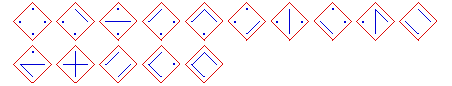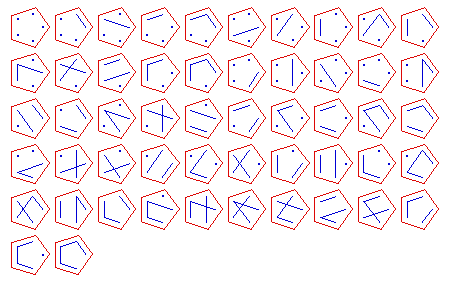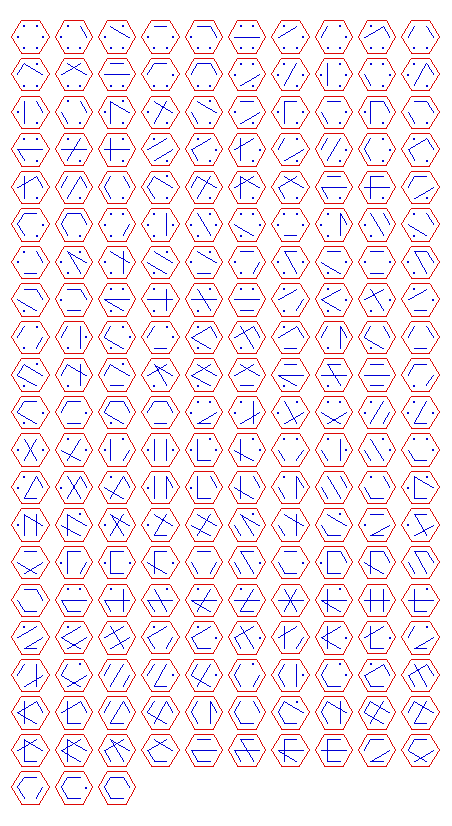# Bell number diagrams

The Bell numbers
(1, 1, 2, 5, 15, 52, 203, 877, 4140, 21147, 115975, 678570, 4213597, ...)
describe the number of ways a set with n elements can be partitioned into disjoint, non-empty subsets.

For example, the set {1, 2, 3} can be partitioned in the following ways:

• {{1}, {2}, {3}}
• {{1, 2}, {3}}
• {{1, 3}, {2}}
• {{1}, {2, 3}}
• {{1, 2, 3}}.

The nth Bell number can be computed using the formula
$\sum_{k=0}^n S_n^{(k)}$
where $S_n^{(k)}$ represents the Stirling numbers of the second kind.

Here are some diagrams representing the different ways the sets can be partitioned: a line connects elements in the same subset, and a point represents a singleton subset.

{1, 2, 3}, 5 partitions:{1, 2, 3, 4}, 15 partitions:{1, 2, 3, 4, 5}, 52 partitions:{1, 2, 3, 4, 5, 6}, 203 partitions:{1, 2, 3, 4, 5, 6, 7}, 877 partitions:

(The GIF image is about 125K, so let me know if you're dying to see it.)